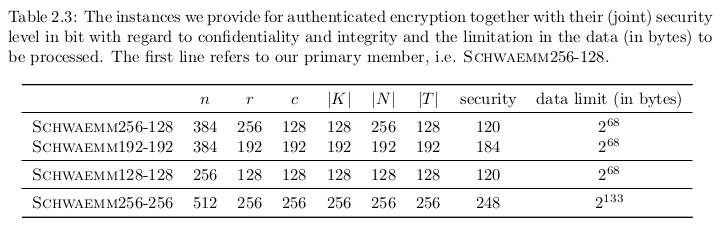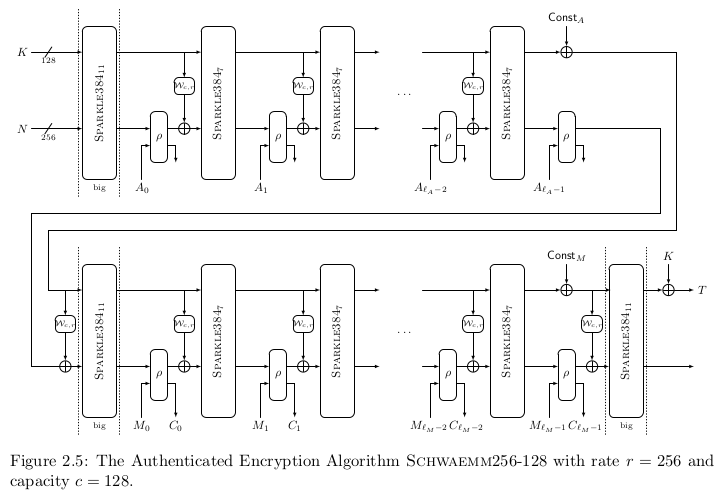# Schwaemm, Authenticated Encryption with Associated Data

Schwaemm is an Authenticated Encryption with Associated Data (AEAD) algorithm that uses the Sparkle permutation in a specific mode. Several versions exist, each providing a specific security level and requiring a permutation operating on a specific block size. The following table summarizes the proposed instances of the family. Here, $$n$$ denotes the size of the permutation (in bits), $$r$$ denotes the rate, $$c$$ denotes the capacity, $$|K|, |N|, |T|$$ denote the sizes of key, nonce and tag respectively.All instances use (a slight variation of) the Beetle mode of operation presented, which is based on the well-known SpongeWrap AEAD mode.

The difference between the instances is the version of the underlying Sparkle permutation (and thus the rate and capacity is different) and the size of the authentication tag. The following figure shows the structure on the example of Schwaemm256-128.